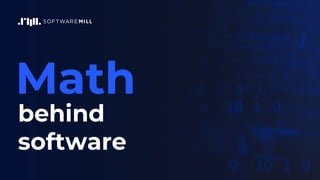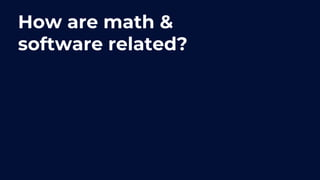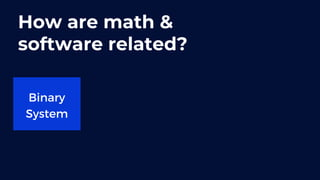Ce diaporama a bien été signalé.
Le téléchargement de votre SlideShare est en cours. ×

# Math Behind Software

Publicité
Publicité
Publicité
Publicité
Publicité
Publicité
Publicité
Publicité
Publicité
Publicité
PublicitéChargement dans…3
×

## Consultez-les par la suite

1 sur 35 Publicité

# Math Behind Software

Be aware: it's mostly theoretical math here!

But math is an essential part of our everyday work as software engineers, so it's worth having a closer look at what the math behind software is.

This presentation is focused on the concept of vacuous truth and how it can impact our work.

The talk opens with an intro on how and where math intersects with software development and moves on to defining vacuous truth exactly and a brief explanation of why calling .allMatch on empty Stream will always return true.

Be aware: it's mostly theoretical math here!

But math is an essential part of our everyday work as software engineers, so it's worth having a closer look at what the math behind software is.

This presentation is focused on the concept of vacuous truth and how it can impact our work.

The talk opens with an intro on how and where math intersects with software development and moves on to defining vacuous truth exactly and a brief explanation of why calling .allMatch on empty Stream will always return true.

Publicité
Publicité

### Math Behind Software

1. 1. Math behind software
2. 2. How are math & software related?
3. 3. How are math & software related? Binary System
4. 4. How are math & software related? Binary System Algorithms
5. 5. How are math & software related? Binary System Algorithms CAP theorem
6. 6. How are math & software related? Binary System Algorithms CAP theorem Monads
7. 7. Why calling allMatch on empty Stream always return true?
8. 8. Why calling allMatch on empty Stream always return true? ...And what does it have to do with math?
9. 9. Vacuous truth
10. 10. Vacuous truth
11. 11. Vacuous truth Is a concept from logic used to describe all statements that are evaluated as true because their base condition (Antecedent) cannot be fulfilled
12. 12. Vacuous truth Is a concept from logic used to describe all statements that are evaluated as true because their base condition (Antecedent) cannot be fulfilled Or in a more mathematical syntax
13. 13. S: P ⇒Q
14. 14. S: P ⇒Q Then, if P is false, S is logically true and considered vacuously true.
15. 15. S: P ⇒Q Then, if P is false, S is logically true and considered vacuously true. It can also be defined with quantificator - for all ∀:
16. 16. S: P ⇒Q Then, if P is false, S is logically true and considered vacuously true. It can also be defined with quantificator - for all ∀: ∀x ∈B : P(x) ⇒Q(x)
17. 17. S: P ⇒Q Then, if P is false, S is logically true and considered vacuously true. It can also be defined with quantificator - for all ∀: ∀x ∈B : P(x) ⇒Q(x) Then, if P(x) is false for all x, our statement is also considered vacuously true.
18. 18. Example If there are any developers in the room next door, then they all work with Haskell.
19. 19. Example If there are any developers in the room next door, then they all work with Haskell. the base condition is: If there are any developers in the room next door
20. 20. Example If there are any developers in the room next door, then they all work with Haskell. the base condition is: If there are any developers in the room next door if the room's empty, the statement is evaluated as vacuously true
21. 21. Haskell devs & mixed syntax
22. 22. Haskell devs & mixed syntax P(x) is our explicit base condition - there are any developers in the room
23. 23. Haskell devs & mixed syntax P(x) is our explicit base condition - there are any developers in the room Q(x) is our 'then' clause - they all work in Haskell
24. 24. Haskell devs & mixed syntax P(x) is our explicit base condition - there are any developers in the room Q(x) is our 'then' clause - they all work in Haskell B is our set - all the people in the room
25. 25. Haskell devs & mixed syntax P(x) is our explicit base condition - there are any developers in the room Q(x) is our 'then' clause - they all work in Haskell B is our set - all the people in the room We can write the example in this form: For all people from the room, if a person is a developer, then they work with Haskell.
26. 26. How exactly do Stream and allMatch fit into this?
27. 27. How exactly do Stream and allMatch fit into this? ∀x ∈B : P(x) ⇒Q(x)
28. 28. Similar behavior in other languages
29. 29. Similar behavior in other languages In Scala: forall, present in many collections
30. 30. Similar behavior in other languages In Scala: forall, present in many collections In JavaScript: every from array prototype
31. 31. Similar behavior in other languages In Scala: forall, present in many collections In JavaScript: every from array prototype In Python & Kotlin: all - which tests if all elements of an array are true, will also return true for empty arrays
32. 32. When hell freezes... Vacuous truth IRL when hell freezes when pigs fly when shrimps learn to whistle when the sun starts rising in the west ...or any other absurdity based on an obviously false or impossible condition
33. 33. Vacuous truth - recap: is a concept from the mathematics field of logic represents logical statements with a false base condition is the reason why allMatch and their counterparts will return true for empty sequences can sometimes occur in real life
34. 34. Thank you! Bartek Żyliński @PaskSoftware softwaremill.com